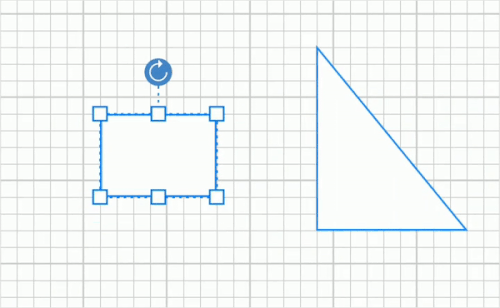# Gridlines in Xamarin Diagram (SfDiagram)

Gridlines are the pattern of lines drawn behind the diagram elements. It provides a visual guidance when dragging or arranging the node on the diagram surface.

## Gridlines visibility

The “ShowGrid” property in PageSettings will show or hide the gridlines. The following code example illustrates how to enable grid visibility.

``````<diagram:SfDiagram x:Name="diagram" >
<diagram:SfDiagram.PageSettings>
<diagram:PageSettings ShowGrid =“true”/>
</diagram:SfDiagram.PageSettings>
</diagram:SfDiagram>``````
``diagram.PageSettings.ShowGrid = true;``

## Customizing gridlines

Grid cell size and gridline color can be modified using the “GridSize” and “GridColor” properties respectively. The following code example illustrates how to customize the grid.

``````<diagram:SfDiagram x:Name="diagram" >
<diagram:SfDiagram.PageSettings>
<diagram:PageSettings GridColor ="Pink” GridSize =“14”/>
</diagram:SfDiagram.PageSettings>
</diagram:SfDiagram>``````
``````diagram.PageSettings.GridColor = Color.Pink;
diagram.PageSettings.GridSize = 14;``````

## Snapping gridlines

Nodes can be aligned and resized easily using gridlines by enabling the snap to grid. The following code example illustrates how to enable the snap to grid.

``````<diagram:SfDiagram x:Name="diagram" >
<diagram:SfDiagram.PageSettings>
<diagram:PageSettings SnapToGrid=”true”/>
</diagram:SfDiagram.PageSettings>
</diagram:SfDiagram>``````
``diagram.PageSettings.SnapToGrid = true;``